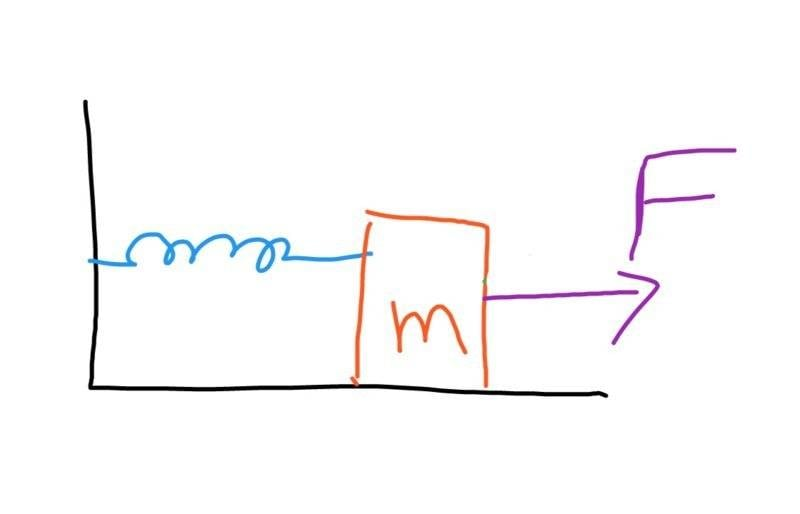# Is F=spring force generated?

Gold MemberIs it necessary that the applied force F is equal to the spring force 'kx'?

#### Attachments

jbriggs444
Homework Helper
Is it necessary that the applied force F is equal to the spring force 'kx'?
Are you trying to invoke Newton's 2nd law or Newton's 3rd law? What, if anything, do either have to say about the situation?

Gold Member
I am not trying to invoke any law
Are you trying to invoke Newton's 2nd law or Newton's 3rd law? What, if anything, do either have to say about the situation?

sophiecentaur
Gold Member
Is the mass stationary?

Gold Member
No
Is the mass stationary?

russ_watters
Mentor
View attachment 232217
Is it necessary that the applied force F is equal to the spring force 'kx'?
The way you've drawn the picture, it looks like F is a separate force applied to the mass, not the spring force. You'll have to tell us what the drawing means - you drew it! (presumably).

sophiecentaur
Gold Member
No
Make the spring as weak as you like. Would F be zero?

Gold Member
Yes,F(on right) is a separate force applied to mass m other than the spring force acting on the left side.
The way you've drawn the picture, it looks like F is a separate force applied to the mass, not the spring force. You'll have to tell us what the drawing means - you drew it! (presumably).

Chestermiller
Mentor
Please write down for us your Newton's 2d law force balance equation on the mass M.

Gold Member
F-kx=ma
Please write down for us your Newton's 2d law force balance equation on the mass M.

Chestermiller
Mentor
F-kx=ma

•navneet9431 and russ_watters
russ_watters
Mentor
Yes,F(on right) is a separate force applied to mass m other than the spring force acting on the left side.
In that case it can have literally any value you choose to give it.

Gold Member
So it means that F=/=kx

Gold Member
But is it possible that F=kx in any condition?

ZapperZ
Staff Emeritus
But is it possible that F=kx in any condition?

If the mass is stationary, or if F is applied in such a way that the mass is moving at a constant velocity. Look at the equation you wrote. What is "a" under these two situations?

Zz.

•navneet9431
Gold Member
Thanks
If the mass is stationary, or if F is applied in such a way that the mass is moving at a constant velocity.

Zz.

But is it possible that F=kx in any condition?

YOU will choose that.

If you choose |F|>|kx|, then m won't be at rest, but will have a constant acceleration rightwards.
If |F| = |kx|, then you have achieved an equilibrium, nothing moves. (In lab, this is how we calculate spring constant, where F=mg)

If |F| < |kx|, then you will have the spring pulling m leftwards.

•sophiecentaur